An Logic binary Adder circuit can add two or more binary bits and gives result as Sum, Carry. It can be used in many applications like BCD (binary coded decimal), Encoder, address decoder, Binary calculation etc.., the basic binary adder circuit classified into two categories they are,

Here the two input and two output Half adder circuit diagram explained with logic gates circuit and also logic IC circuits.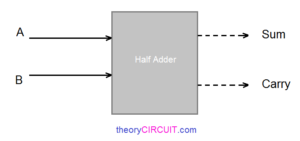The Half adder will take two inputs Named as A, B then it will give two outputs named as Sum, Carry.

• Sum   = A ⊕ B
• Carry = A . B

The result Sum is exclusive addition between A, B, then result Carry is AND logic operation between A, B.

## Half Adder Logic Gate diagram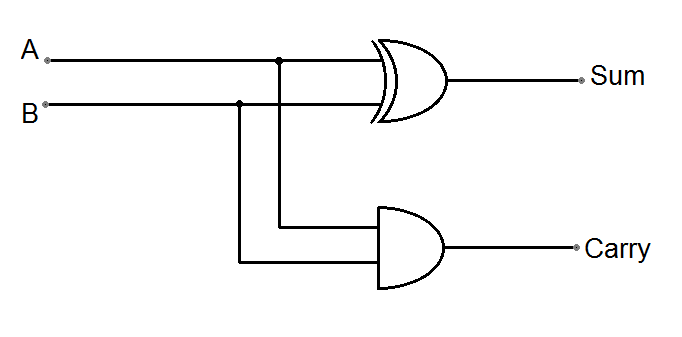Two input XOR gate, two input AND gate forms the Half Adder logic circuit. Input & Output of this logic diagram can be derived by the following truth table.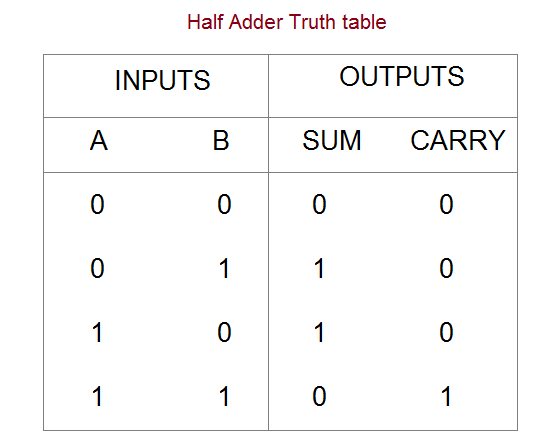When both inputs are low then sum and carry will be logic low (0), If any one input is high then Sum will be logic high (1) and carry will be logic low (0). When both inputs are high then Sum becomes logic low (0) and Carry becomes logic high (1).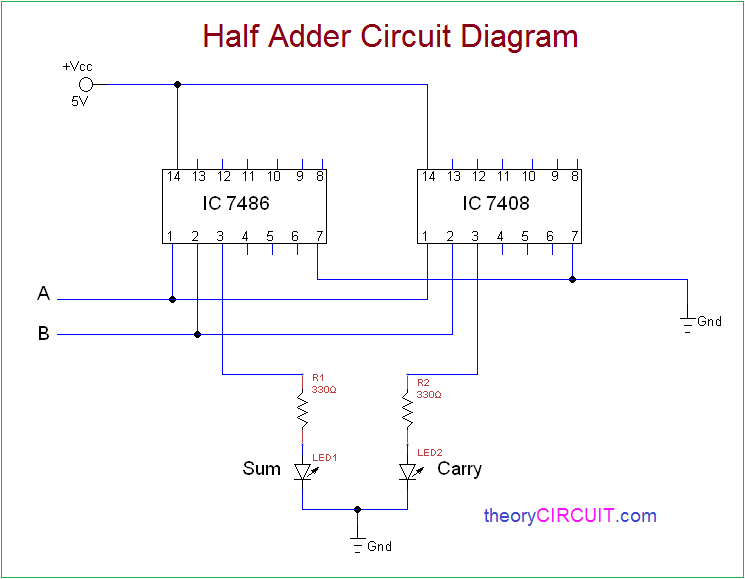Construction & Working

Here XOR gate IC 7486 and Logic AND gate IC 7408 are used to construct the half adder circuit, both are quad 2 input logic gate IC. First connect Vcc +5V and GND supply to logic IC, Input Points A, B are connected to the XOR gate input then AND gate input, both IC will give output at pin 3 hence the XOR gate output connected to the LED1 through R1 resistor (Sum output indicator). AND gate output connected to the LED2 through R2 resistor (Carry output indicator). When the LED glows it represents logic High (1), if the LED stays OFF then it represents logic LOW (0).

IC 7486 – IC 7408 pinout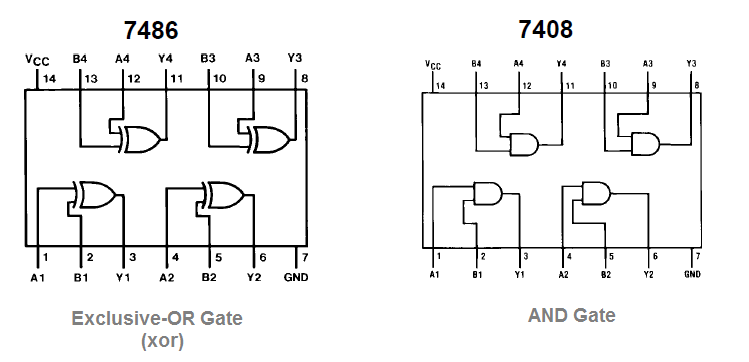Note:-

* Connect Input A, B to +Vcc for logic High (1) input.

* Connect Input A, B to  GND for logic Low (0) input.

* Some times open A, B terminal represents logic High.

## One thought on “Half Adder Circuit Diagram”

1.Waseem Asghar says:

Excellent work
Well done job